Courses

# NCERT Solutions(Part- 2)- Mensuration Class 8 Notes | EduRev

## Class 8 : NCERT Solutions(Part- 2)- Mensuration Class 8 Notes | EduRev

The document NCERT Solutions(Part- 2)- Mensuration Class 8 Notes | EduRev is a part of Class 8 category.
All you need of Class 8 at this link: Class 8

Question: We know that parallelogram is also a quadrilateral. Let us also split such a quadrilateral into two triangles, find their areas and hence that of the parallelogram. Does this agree with the formula that you know already?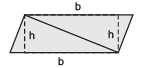Solution: The diagonal BD of quadrilateral ABCD is joined and it divides the quadrilateral into two triangles.

Now,

Area of quadrilateral ABCD = Area of D ABD + Area of D BCD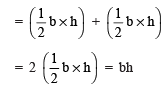Infact ABCD is a parallelogram.

∴ Area of a parallelogram ABCD = b * h

Area of a parallelogram = Base * Height

We know that a parallelogram can also be a trapezium. We already know that

Area of trapezium ABCD = 1/2 (Sum of parallel sides) * [Perpendicular distance between the parallel sides]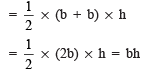or Area of parallelogram ABCD = bh.

Yes, the above relation agrees with formula that we know already.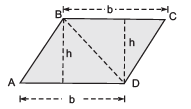Question: A parallelogram is divided into two congruent triangles by drawing a diagonal across it. Can we divide a trapezium into two congruent triangles?

Solution: No, a trapezium cannot be divided into two congruent triangles.

Let ABCD be a rhombus. Therefore, its diagonals are perpendicular to each other.

Area of rhombus ABCD = Area of Δ ACD + Area of Δ ABC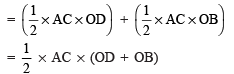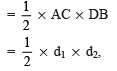where d1 and d2 are the diagonals of the rhombus.

Thus, the area of a rhombus =1/2 * The product of its diagonals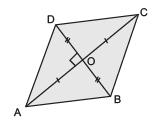Note: Splitting a quadrilateral into triangles is called triangulation.

Example 2. Find the area of a rhombus whose diagonals are 12 cm and 9.2 cm.

Solution: Let d1 and d2 be the diagonals of the rhombus.

∴ d1 = 12 cm and d2 = 9.2 cm

∵ Area of rhombus =1/2  * d1 * d2

∴ Area of the given rhombus =1/2 * 12 * 9.2 cm2

= 6 * 9.2 cm2 = 55.2 cm2

Question: Find the area of these quadrilaterals: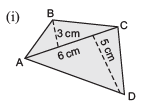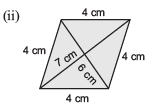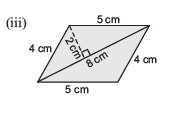Solution:

= 1/2 * AC * [Sum of perpendiculars on AC from opposite vertices]

= 1/2 * 6 cm * [3 cm + 5 cm]

= 1/2 * 6 cm * 8 cm = 3 cm * 8 cm = 24 cm2

(ii) The given figure is a rhombus having d1 = 7 cm and d2 = 6 cm.

∴  Area of the given rhombus =1/2 * Product of diagonals

= 1/2 * d1 * d2

= 1/2 *7 cm * 6 cm

= 7 cm * 3 cm = 21 cm2

(iii) The given figure is a parallelogram. Its diagonal divides it in totwo congruent triangles.

∴ Area of the parallelogram = 2 * [Area of one of the triangles]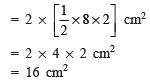Area of a Polygon

To find area of polygons, we divide them into shapes, for which we have a formula for the area. First we find the areas of various parts and then add them to get the area of given polygon.

Question 1. Divide the following polygons into parts (triangles and trapezium) to find out its area.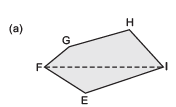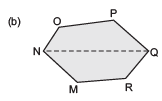Solution:

(a) We draw perpendiculars from opposite vertices on FI, i.e. GL ⊥ FI, HM ⊥ FI and EN ⊥ FI

Area of the polygon EFGHI

= ar (Δ GFL) + ar (trapezium GLMH) + ar (Δ HMI) + ar (Δ NEI) + ar (Δ EFN)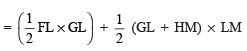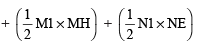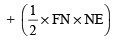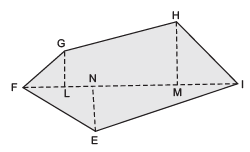(b) NQ is a diagonal. Draw OA ⊥ NQ, MB ⊥ NQ, PC ⊥ NQ and RD ⊥ NQ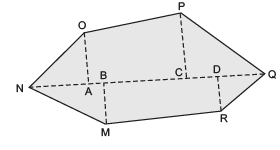∴ Area of polygon OPQRMN = ar (Δ OAN) + ar (trap. CPOA) + ar (Δ PCQ) + ar (Δ RDQ)+ ar (trap. MBDR) + ar (Δ MBN)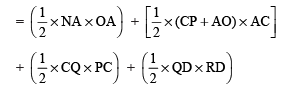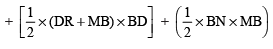Question 2. Fill in the blanks.

Polygon ABCDE is divided into parts as shown below. Find its area if AD = 8 cm, AH = 6 cm, AG = 4 cm, AF = 3 cm and perpendiculars BF = 2 cm, CH = 3 cm, EG = 2.5 cm.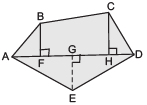Area of polygon ABCDE = Area of ΔAFB + …

Area of Δ AFB =1/2 * AF * BF = 1/2 * 3 * 2 = …

Area of trapezium FBCH =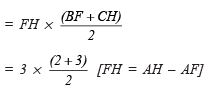So, the area of polygon ABCDE = …

Solution: Area of polygon ABCDE = Area of Δ AFB + Area of trapezium FBCH + Area of Δ CHD + Area of Δ ADE

Area of D AFB = 1/2 * AF * BF

= 1/2 * 3 * 2 = 3 cm2

Area of trapezium FBCH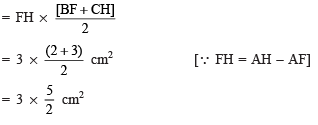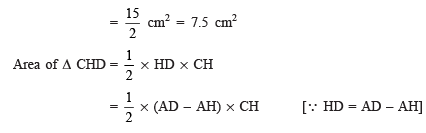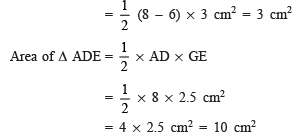So, the area of polygon ABCD = 3 cm2 + 7.5 cm2 + 3 cm2 + 10 cm2 = 23.5 cm2

Question 3. Find the area of polygon MNOPQR if MP = 9 cm, MD = 7 cm MC = 6 cm, MB = 4 cm, MA = 2 cm. NA, OC, QD and RB are perpendiculars to diagonal MP.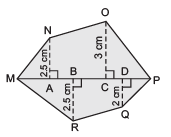Solution: Area of polygon MNOPQR = ar (Δ MAN) + ar (trap. ACON) + ar (Δ OCP) + ar (Δ PDQ) + ar (trap. DBRQ) + ar Δ RBM)

∵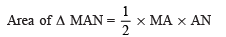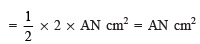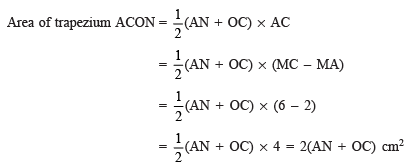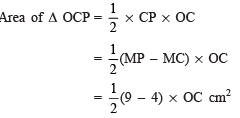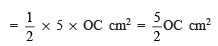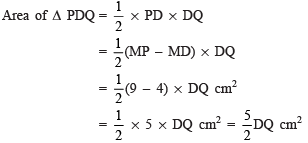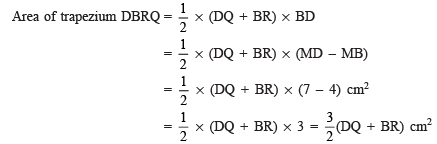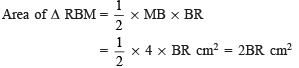∴ Area of polygon MNOPQR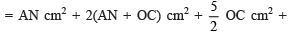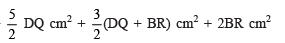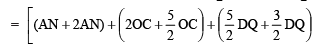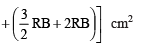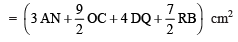Offer running on EduRev: Apply code STAYHOME200 to get INR 200 off on our premium plan EduRev Infinity!

,

,

,

,

,

,

,

,

,

,

,

,

,

,

,

,

,

,

,

,

,

;